Courses

# ICAI Notes 2.1 - Law of Demand and Elasticity of Demand CA Foundation Notes | EduRev

## CA Foundation : ICAI Notes 2.1 - Law of Demand and Elasticity of Demand CA Foundation Notes | EduRev

The document ICAI Notes 2.1 - Law of Demand and Elasticity of Demand CA Foundation Notes | EduRev is a part of the CA Foundation Course Economics for CA CPT.
All you need of CA Foundation at this link: CA Foundation

Learning Objectives

• understand the meaning of demand.
• understand what determines demand.
• get an insight into the law of demand.
• understand the difference between movement in the demand curve and shift of the demand curve.
• know various types of elasticity of demand.

1.0 MEANING OF DEMAND

The concept ‘demand’ refers to the quantity of a good or service that consumers are willing and able to purchase at various prices during a period of time. It is to be noted that demand in Economics is something more than desire to purchase though desire is one element of it. A beggar, for instance, may desire food, but due to lack of means to purchase it, his demand is not effective. Thus effective demand for a thing depends on
(i) desire
(ii) means to purchase and
(iii) on willingness to use those means for that purchase. Unless demand is backed by purchasing power or ability to pay, it does not constitute demand. Two things are to be noted about quantity demanded. One is that quantity demanded is always expressed at a given price. At different prices different quantities of a commodity are generally demanded. The second thing is that quantity demanded is a flow. We are concerned not with a single isolated purchase, but with a continuous flow of purchases and we must therefore express demand as so much per period of time – one thousand dozens oranges per day, seven thousand dozen oranges per week and so on.

In short “By demand, we mean the various quantities of a given commodity or service which consumers would buy in one market in a given period of time, at various prices, or at various incomes, or at various prices of related goods”.

1.1 WHAT DETERMINES DEMAND?

There are a number of factors which influence household demand for a commodity. Important among these are :

(i) Price of the commodity : Ceteris paribus i.e. other things being equal, the demand of a commodity is inversely related to its price. It implies that a rise in price of a commodity brings about a fall in its purchase and vice-versa. This happens because of income and substitution effects.

(ii) Price of related commodities : Related commodities are of two types :
(a) complementary goods and
(b) competing goods or substitutes.

Complementary goods are those goods which are consumed together or simultaneously.

For example, tea and sugar, automobiles and petrol, pen and ink are used together. When commodities are complements, a fall in the price of one (other things being equal) will cause the demand of the other to rise.

For examplea fall in the price of cars would lead to a rise in the demand for petrol. Similarly, a fall in the price of pens, will cause a rise in the demand for ink. The reverse will be the case when the price of a complement rises. Competing goods or substitutes are those goods which can be used with ease in place of one another. For example, tea and coffee, ink pen and ball pen, are substitutes for each other and can be used in place of, one another easily. When goods are substitutes, a fall in the price of one (ceteris paribus) leads to a fall in the quantity demanded of its substitutes. For example, if the price of tea falls, people will try to substitute it for coffee and demand more of it and less of coffee i.e. the demand for tea will rise and that of coffee fall.

(iii) Level of income of the household : Other things being equal, the demand for a commodity depends upon the money income of the household. In most cases, the larger the average money income of the household, the larger is the quantity demanded of a particular good. However, there are certain commodities for which quantities demanded decrease with an increase in money income. These goods are called inferior goods. Even in the case of other goods, the response of quantities demanded to changes in their prices is not of same proportions. If goods are such that they satisfy the basic necessities (food, clothing, shelter) of life, a change in their prices although will cause an increase in demand for these necessities this increase will be less than proportionate to the increase in income. This is because as people become richer, there is a relative decline in importance of food and other non durable goods in the over all consumption pattern and a rise in importance of durable goods such as a TV, car, house etc.

(iv) Tastes and preferences of consumers : The demand for a commodity also depends upon tastes and preferences of consumers and changes in them over a period of time. Goods which are more in fashion command higher demand than goods which are out of fashion. Consumers may even discard a good even before it is fully utilised and prefer another good which is in fashion. For example, there is a greater demand for coloured television and more and more people are discarding their black and white television even though they could have still used it for some more years.

‘Demonstration effect’ plays an important role in affecting the demand for a product. An individual’s demand for colour television may be affected by his seeing one in neighbour’s or friend’s house, either because he likes what he sees or because he figures out that if his neighbour or friend can afford it, he too can. A person may develop a taste or preference for wine after tasting some, but he may also develop it after discovering that serving it enhances his prestige. In any case, people have tastes and preferences and these change, sometimes due to external and sometimes due to internal causes.

(v) Other factors : Apart from the above factors, the demand for a commodity depends upon the following factors :

(a) Size of population : Generally, larger the size of population of a country or a region, greater is the demand for commodities in general.

(b) Composition of population : If there are more old people in a region, the demand for spectacles, walking sticks, etc. will be high. Similarly, if the population consists of more of children, demand for toys, baby foods, toffees, will be more.

(c) Distribution of income : The wealth of a country may be so distributed that there are a few very rich people while the majority are very poor. Under such conditions the propensity to consume of the country will be relatively less, for the propensity to consume of the rich people is less than that of the poor people. Consequently, the demand for consumer goods will be comparatively less. If the distribution of income is more equal, then the propensity to consume of the country as a whole will be relatively high indicating higher demand for goods. Apart from above, factors such as class, group, education, marital status, consumer’s expectations with regard to future price and weather conditions, also play an important role in influencing household demand.

1.2 LAW OF DEMAND

The law of demand is one of the most important laws of economic theory. According to law of demand, other things being equal, if the price of a commodity falls, the quantity demanded of it will rise and if the price of a commodity rises, its quantity demanded will decline.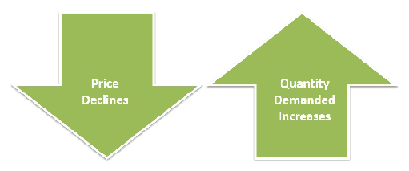Thus, there is an inverse relationship between price and quantity demanded, other things being same. The other things which are assumed to be equal or constant are the prices of related commodities, income of consumers, tastes and preferences of consumers, and such other factors which influence demand. If these factors which determine demand also undergo a change, then the inverse price-demand relationship may not hold good. For example, if incomes of consumers increase, then an increase in the price of a commodity, may not result in a decrease in the quantity demanded of it. Thus the constancy of these other factors is an important assumption of the law of demand. The law of demand may be illustrated with the help of a demand schedule and a demand curve.

1.2.0 Demand Schedule : To illustrate the relation between the quantity of a commodity demanded and its price, we may take hypothetical data for prices and quantities of a commodity X.

Table 1 : Demand schedule of an individual consumer

 Price (Rs.) Quantity demanded A 5 10 B 4 15 C 3 20 D 2 35 E 1 60

When price of commodity X is Rs. 5 per unit, a consumer purchases 10 units of the commodity. When the price falls to Rs. 4, he purchases 15 units of the commodity. Similarly, when the price further falls, quantity demanded by him goes on rising until at price Re. 1, the quantity demanded by him rises to 60 units. The above table depicts an inverse relationship between price and quantity demanded as the price of the commodity X goes on rising, its demand goes on falling.

Demand curve : We can now plot the data from Table 1 on a graph with price on the vertical axis and quantity on the horizontal axis. In Fig. 1, we have shown such a graph and plotted the five points corresponding to each price-quantity combination shown in Table 1. Point A, shows the same information as the first row of Table 1, that at Rs. 5 per unit, only 10 units of X will be demanded. Point E shows the same information as does the last row of the table, when the price is Re. 1, the quantity demanded will be 60 units.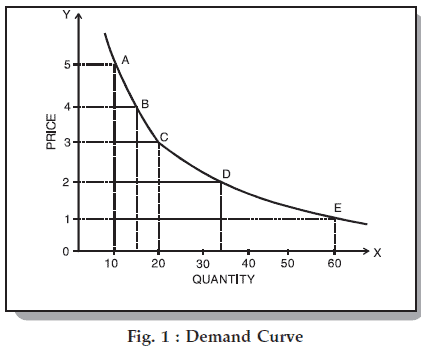We now draw a smooth curve through these points. The curve is called the demand curve for commodity ‘X’. The curve shows the quantity of ‘X’ that a consumer would like to buy at a each price; its downward slope indicates that the quantity of ‘X’ demanded increases as its price falls. Thus the downward sloping demand curve is in accordance with the law of demand which as stated above, describes an inverse price-demand relationship.

1.2.1 Market Demand Schedule : When we add up the various quantities demanded by the number of consumers in the market we can obtain the market demand schedule. How the summation is done is illustrated in Table 2. Suppose there are three individual buyers of the goods in the market. The Table 2 shows their individual demands at various prices.

Table 2 : Market Demand Schedule

 Quantity demanded by
 Price (Rs.) P Q R Total market demand 5 10 8 12 30 4 15 12 18 45 3 20 17 23 60 2 35 25 40 100 1 60 35 45 140

When we add quantities demanded at each price by consumers P, Q, R we get total market demand. Thus when price is Rs. 5 per unit, the demand for commodity ‘X’ in the market is 30 units (i.e. 10+8+12). When price falls to Rs. 4, market demand is 45 units. At Re. 1, 140 units are demanded in the market. The market demand schedule also indicates inverse relationship between price and quantity demanded of ‘X’.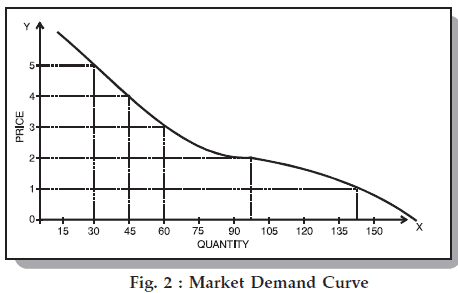Market Demand Curve : If we plot market demand schedule on a graph we get market demand curve. Figure 2 shows market demand curve for commodity ‘X’. The market demand curve, like individual demand curve, slopes downwards to the right because it is nothing but lateral summation of individual demand curves. Besides, as the price of the good falls, it is very likely that new buyers will enter the market which will further raise the quantity demanded of the goods.

1.2.2 Rationale for the Law of Demand : Why does demand curve slope downwards?

(1) When the price of a commodity falls, it becomes relatively cheaper than other commodities. It induces consumers to substitute the commodity whose price has fallen for other commodities which have now become relatively expensive. The result is that total demand for the commodity whose price has fallen increases. This is called substitution effect.

(2) When the price of a commodity falls, the consumer can buy the same quantity of the commodity with lesser money or he can buy more of the same commodity with the same money. In other words, as a result of fall in the price of the commodity, consumer’s real income or purchasing power increases. This increase in the real income induces him to buy more of that commodity. Thus, demand for that commodity (whose price has fallen) increases. This is called income effect.

(3) When the price of a commodity falls, more consumers start buying it because some of those who could not afford to buy it previously may now afford to buy it. This raises the number of consumers of a commodity at a lower price and hence the demand for the commodity in question.

1.2.3 Exceptions to the Law of Demand : According to the law of demand, more of a commodity will be demanded at lower prices than at higher prices, other things being equal. The law of demand is valid in most of the cases; however there are certain cases where this law does not hold good. The following are the important exceptions to the law of demand.

(i) Conspicuous goods : Articles of prestige value or snob appeal or articles of conspicuous consumption are demanded only by the rich people and these articles become more attractive if their prices go up. Such articles will not conform to the usual law of demand. This was found out by Veblen in his doctrine of “Conspicuous Consumption” and hence this effect is called Veblen effect or prestige goods effect. Veblen effect takes place as some consumers measure the utility of a commodity by its price i.e., if the commodity is expensive they think that it has got more utility. As such, they buy less of this commodity at low price and more of it at high price. Diamonds are often given as example of this case. Higher the price of diamonds, higher is the prestige value attached to them and hence higher is the demand for them.

(ii) Giffen goods : Sir Robert Giffen, an economist, was surprised to find out that as the price of bread increased, the British workers purchased more bread and not less of it. This was something against the law of demand. Why did this happen? The reason given for this is that when the price of bread went up, it caused such a large decline in the purchasing power of the poor people that they were forced to cut down the consumption of meat and other more expensive foods. Since bread even when its price was higher than before was still the cheapest food article, people consumed more of it and not less when its price went up.

Such goods which exhibit direct price-demand relationship are called ‘Giffen goods’. Generally those goods which are considered inferior by the consumers and which occupy a substantial place in consumer’s budget are called ‘Giffen goods’. Examples of such goods are coarse grains like bajra, low quality of rice and wheat etc.

(iii) Conspicuous necessities : The demand for certain goods is affected by the demonstration effect of the consumption pattern of a social group to which an individual belongs. These goods, due to their constant usage, have become necessities of life. For example, in spite of the fact that the prices of television sets, refrigerators, coolers, cooking gas etc. have been continuously rising, their demand does not show any tendency to fall.

(iv) Future expectations about prices : It has been observed that when the prices are rising, households expecting that the prices in the future will be still higher, tend to buy larger quantities of the commodities. For example, when there is wide-spread drought, people expect that prices of foodgrains would rise in future. They demand greater quantities of foodgrains as their price rise. But it is to be noted that here it is not the law of demand which is invalidated but there is a change in one of the factors which was held constant while deriving the law of demand, namely change in the price expectations of the people.

(v) The law has been derived assuming consumers to be rational and knowledgeable about market-conditions :  However, at times consumers tend to be irrational and make impulsive purchases without any cool calculations about price and usefulness of the product and in such contexts the law of demand fails.

(vi) Demand for Necessaries: The law of demand does not apply much in the case of necessaries of life. Irrespective of price changes, people have to consume the minimum quantities of necessary commodities. Similarly, in practice, a household may demand larger quantity of a commodity even at a higher price because it may be ignorant of the ruling price of the commodity. Under such circumstances, the law will not remain valid.

(vii) Speculative goods: In the speculative market, particularly in stock and shares, more will be demanded when the prices are rising and less will be demanded when the price declines. The law of demand will also fail if there is any significant change in other factors on which demand of a commodity depends. If there is a change in income of the household, or in prices of the related commodities or in tastes and fashion etc. the inverse demand and price relation may not hold good.

1.3 EXPANSION AND CONTRACTION IN DEMAND

The demand schedule, demand curve and the law of demand all show that when the price of a commodity falls its quantity demanded increases, other things being equal. When as a result of decrease in price, the quantity demanded increases, in Economics, we say that there is an expansion of demand and when as a result of increase in price, quantity demanded decreases we say that there is contraction of demand. For example, suppose the price of apples at any time is Rs. 10 per kilogram and a consumer buys one kilogram at that price. Now, if other things such as income, prices of other goods and tastes of the consumers remain same but the price of apples falls to Rs. 8 per kilogram and the consumer now buys two kilograms of apples, we say that there is a change in quantity demanded or there is an expansion of demand. On the contrary, if the price of apples rises to Rs. 15 per kilogram and consumer buys only half a kilogram, we say that there is a contraction of demand.

The phenomena of expansion and contraction in demand are shown in Figure 3. The figure shows that when price is OP quantity demanded is OM, given other things equal. If as a result of increase in price (OP”), the quantity demanded falls to OL we say there is ‘a fall in quantity demanded’ or ‘contraction of demand’ or ‘an upward movement along the same curve’. Similarly, as a result of fall in price to OP’ the quantity demanded rises to ON, we say that there is ‘expansion of demand’ or ‘a rise in quantity demanded’ or ‘a downward movement on the same demand curve.’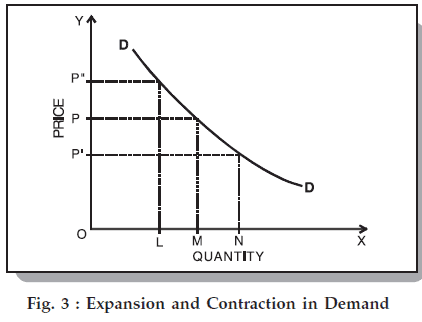1.4 INCREASE AND DECREASE IN DEMAND

Till now we have assumed that other determinants remain constant when we are analysing demand for a commodity. It should be noted that expansion and contraction in demand take place as a result of changes in the price while all other determinants of price viz. income, tastes, propensity to consume and price of related goods remain constant. These other factors remaining constant means that the position of the demand curve remains the same and the consumer moves downwards or upwards on it.What happens if there is a change in, consumers’ tastes and preferences, income, the prices of the related goods or other factors on which demand depends? Let us consider the demand for commodity X :

Table 3 shows for our example of commodity X, the possible effect of an increase in income of the consumer.

Table 3 : Two demand schedules for commodity X

 Price (Rs.) Quantity of ‘X’ demanded when average household income is Rs. 4,000 per month Quantity of ‘X’ demanded when average household income is Rs. 5,000 per month A 5 10 15 A1 B 4 15 20 B1 C 3 20 25 C1 D 2 35 40 D1 E 1 60 65 E1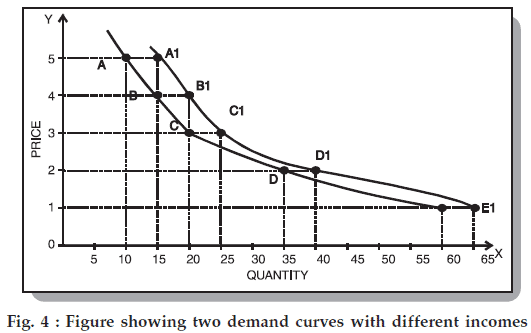These new data are plotted in Figure 4 as demand curve D’D’ along with the original demand curve DD. We say that the demand curve for X has shifted [in this case it has shifted to right]. The shift from DD to D’D’ indicates an increase in the desire to purchase ‘X’ at each possible price. For example, at the price of Rs. 4 per unit, 15 units are demanded when average household income is Rs. 4,000 per month. When the average household income rises to Rs. 5,000 per month, 20 units of X are demanded at price Rs. 4. A rise in income thus shifts the demand curve to the right, whereas a fall in income will have the opposite effect of shifting the demand curve to the left.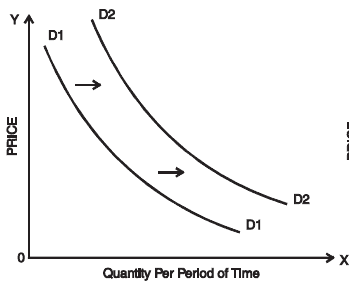Fig. 5(a) : Rightward shift in the demand Curve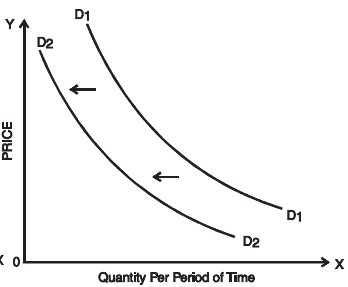Fig. 5(b) : Leftward shift in the demand curve.

(i) A rightward shift in the demand curve : (when more is demanded at each price) can be caused by a rise in income, a rise in the price of a substitute, a fall in the price of a complement, a change in tastes in favour of this commodity, an increase in population, and a redistribution of income to groups who favor this commodity.

(ii) A leftward shift in the demand curve : (when less is demanded at each price) can be caused by a fall in income, a fall in the price of a substitute, a rise in the price of a complement, a change in tastes against this commodity, a decrease in population, and a redistribution of income away from groups who favour this commodity.

1.5 MOVEMENTS ALONG DEMAND CURVE VS. SHIFT OF CURVE

It is important in Economics to make a distinction between a movement along a demand curve and a shift of the whole demand curve.

A movement along the demand curve indicates changes in the quantity demanded because of price changes, other factors remaining constant. A shift of the demand curve indicates that there is a change in demand at each possible price because one or more other factors, such as incomes, tastes or the price of some other goods, have changed.

Thus, when an economist speaks of an increase or a decrease in demand, he refers to a shift of the whole curve because one or more of the factors which were assumed to remain constant earlier have changed. When the economist speaks of change in quantity demanded he means movement along the same curve (i.e., expansion or contraction of demand) which has happened due to fall or rise in price of the commodity.

In short ‘change in demand’ represents shift of the demand curve to right or left resulting from changes in factors such as income, tastes, prices of other goods etc. and ‘change in quantity demanded’ represents movement upwards or downwards on the same demand curve resulting from a change in price of the commodity.

1.6 ELASTICITY OF DEMAND

Till now we were concerned with the direction of the changes in prices and quantities demanded. Now we will try to measure these changes or to say we will try to answer the question “by how much”?

Consider the following situations :

(1) As a result of a fall in the price of radio from Rs. 500 to Rs. 400, the quantity demanded increases from 100 radios to 150 radios.

(2) As a result of fall in the price of wheat from Rs. 10 per kilogram to Rs. 9 per kilogram the demand increases from 500 kilograms to 520 kilograms.

(3) As a result of fall in the price of salt from Rs. 3 per kilogram to Rs. 2.50, the quantity demanded increases from 1000 kilogram to 1005 kilograms.

What do you notice? You notice that as a result of fall in the price of radios, the demand for radios increases. But same is the case with wheat and salt. Thus we can say that demand for the radios, wheat and salt all respond to price changes. Then where is the difference? The difference lies in the degree of response of demand which can be found out by comparing percentage changes in prices and quantities demanded. Here lies the concept of elasticity.

Definition : Elasticity of demand is defined as the responsiveness of the quantity demanded of a good to changes in one of the variables on which demand depends or we can say that it is the percentage change in quantity demanded divided by the percentage in one of the variables on which demand depends. These variables are price of the commodity, prices of the related commodities, income of the consumers and other various factors on which demand depends. Thus we have price elasticity, cross elasticity, elasticity of substitution and income elasticity. It is to be noted that when we talk of elasticity of demand, unless and until otherwise mentioned, we talk of price elasticity of demand. In other words, it is price elasticity of demand which is usually referred to as elasticity of demand.

1.6.0 Price Elasticity : Price elasticity of demand expresses the response of quantity demanded of a good to a change in its price, given the consumer’s income, his tastes and prices of all other goods. In other words, it is measured as percentage change in quantity demanded divided by the percentage change in price, other things remaining equal. That is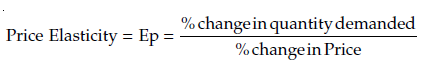Or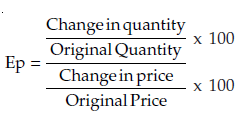Or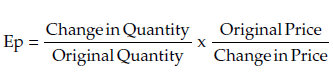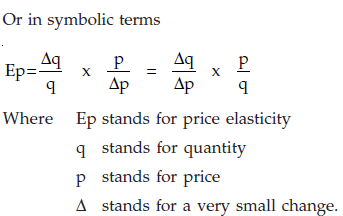Strictly speaking the value of price elasticity varies from minus infinitity to approach zero from the negative sign,  because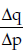has a negative sign. In other words, since price and quantity are inversely related (with a few exceptions) price elasticity is negative. But for the sake of convenience, we ignore the negative sign and consider only the numerical value of the elasticity.

Thus if a 1% change in price leads to 2% change in quantity demanded of good A and 4% change in quantity demanded of good B, then we get elasticity of A and B as 2 and 4 respectively, showing that demand for B is more elastic or responsive to price changes than A. Had we correct. Hence by convention we take absolute value of price elasticity and draw conclusions. A numerical example for the price elasticity of demand:

The price of a commodity decreases from Rs.6 to Rs. 4 and quantity demanded for good increases from 10 units to 15 units. Find the coefficient of price elasticity.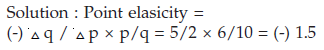Point elasticity : In point elasticity, we measure elasticity at a given point on a demand curve. Point elasticity makes use of derivative rather than finite changes in price and quantity. It may be defined as :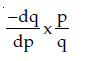where dq/dp is the derivative of quantity with respect to price at a point on the demand curve, and p and q are the price and quantity at that point.

It is to be noted that elasticity is different at different points on the same demand curve. Given a straight line demand curve tT, point elasticity at any point say R can be found by using formula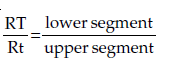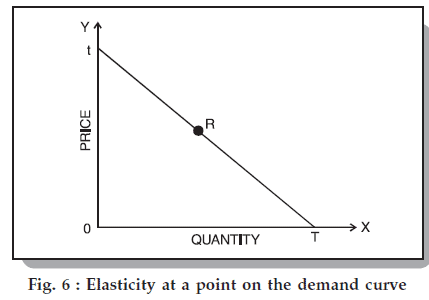Using the above formula we can get elasticity at various points on the demand curve.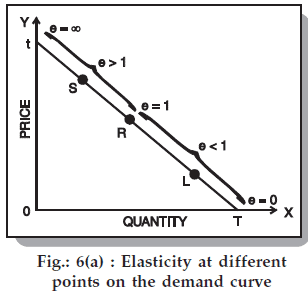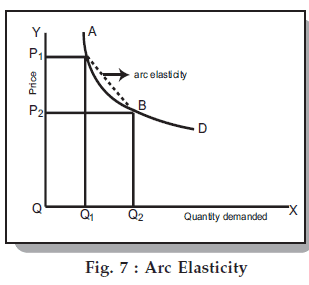Thus we see that as we move from T towards t, elasticity goes on increasing. At the mid-point it is equal to one, at t it is infinity and at T it is zero. Arc-elasticity : When the price change is some what larger or when price elasticity is to be found between the two prices [or two points on the demand curve say A and B in figure 7], the question arise which price and quantity should be taken as base. This is because elasticity  found by using original price and quantity figures as base will be different from the one derived by using new price and quantity figures. Therefore, in order to avoid confusion, generally averages of the two prices and quantities are taken as (i.e. original and new) base. The arc elasticity can be found out by using the formula :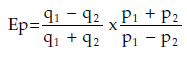where p1, q1 are the original price and quantity and p2, q2 are the new ones. Thus if we have to find elasticity of radios between : p1= Rs. 500 q1 = 100

p2 = Rs. 400 q2 = 150

We will use the formula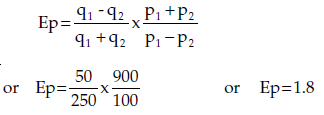Interpreting numerical values of elasticity of demand

The numerical value of elasticity of demand can assume any value between zero and infinity. Elasticity is zero, if there is no change at all in quantity demanded when price changes i.e. when quantity demanded does not respond to a price change.

Elasticity is one, or unitary, if the percentage change in quantity demanded is equal to the percentage change in price. Elasticity is greater than one when the percentage change in quantity demanded is greater than the percentage change in price. In such a case, demand is said to be elastic. Elasticity is less than one when the percentage change in quantity demanded is less than the percentage change in price. In such a case demand is said to be inelastic.

Elasticity is infinite, when some ‘small price reduction raises the demand from zero to infinity. Under such a case consumers will buy all that they can obtain of the commodity at some price. If there is a slight increase in price, they would not buy anything from the particular seller. This type of demand curve is found in perfectly competitive market.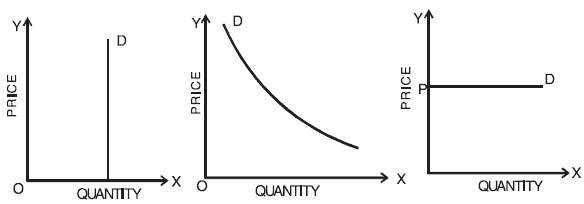Fig. 8 : Demand curve of zero, unitary, and infinite elasticity

Table 4 : Elasticity measures, meaning and nomenclature

 Numerical measure of elasticity Verbal description Terminology Zero Quantity demanded does not change as price changes Perfectly (or completely) inelastic Greater than zero, but less than one Quantity demanded changes by a  smaller percentage than does price Inelastic One Quantity demanded changes by exactly the same percentage as does price Unit elasticity Greater than one, but less than infinity Quantity demanded changes by  a larger percentage than does price Elastic Infinity Purchasers are prepared to buy all they can obtain at some price and  none at all at an even slightly higher price Perfectly (or infinitely) elastic

Now that we are able to classify goods according to their price elasticity, let us see whether the goods which we considered in our example on page 39, are price elastic or inelastic.

 Sl. No. Name of the Commodity Calculation of Elasticity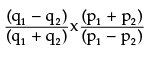Nature of Elasticity 1. Radios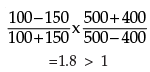Elastic 2. Wheat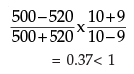Inelastic 3. Common Salt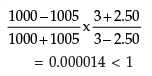Inelastic

.

What do we note in the above hypothetical example? We note that demand for radios is quite elastic, while demand for wheat is quite inelastic and demand for salt is almost same even after a reduction in price.

Generally, in real world situation also, we find that demand for goods like radios, TVs, refrigerators, fans, etc. is elastic, demand for goods like wheat and rice is inelastic, and demand for salt is highly inelastic or perfectly inelastic. Why do we find such a difference in the behaviour of consumers vis-a-vis different commodities? We shall explain later at length those factors which are responsible for the differences in elasticity of demand of various goods. First we will consider another method of calculating price-elasticity which is called total outlay method.

Total Outlay Method of Calculating Price Elasticity : The price elasticity of demand for a commodity and the total expenditure or outlay made on it are greatly related to each other. By analysing the changes in total expenditure or outlay we can know the price elasticity of demand for the good. However, it should be noted that by this method we can only say whether a good is elastic or inelastic; we can not find out the exact coefficient of elasticity.

When as a result of the change in price of a good, the total expenditure on the good remains the same, the price elasticity for the good is equal to unity. This is because total expenditure made on the good can remain the same only if the proportional change in quantity demanded is equal to the proportional change in price. Thus if there is a 100% increase in price of a good and if the price elasticity is unitary, total expenditure of the buyer on the good will remain unchanged.

When as a result of increase in price of a good, the total expenditure made on the good increases or when as a result of decrease in price, the total expenditure made on the good falls, we say that price elasticity of demand is less than unity. In our example of wheat, as a result of fall in price of wheat from Rs. 10 per kg. to Rs. 9 per kg., the total outlay or expenditure falls from 5,000 (10 x 500) to Rs. 4,680 (9 x 520) indicating inelastic demand for wheat. Similarly we can show that as a result of increase in price of wheat from Rs. 9 to Rs. 10 per kg. the total outlay increase from Rs. 4,680 to Rs. 5,000 indicating inelastic demand for wheat.

Determinants of Price Elasticity of Demand : In the above Section we have explained what is price elasticity and how it is measured. Now an important question is : what are the factors which determine whether the demand for a good is elastic or inelastic? We will consider the following important determinants of price elasticity.

(1) Availability of substitutes : One of the most important determinants of elasticity is the degree of availability of close substitutes. Some commodities like butter, cabbage, Maruti, Coca Cola, have close substitutes – margarine, other green vegetables, Santro or other cars, Pepsi or any other cold drink. A change in price of these commodities, the prices of the substitutes remaining constant, can be expected to cause quite substantial substitution – a fall in price leading consumers to buy more of the commodity in question and a rise in price leading consumers to buy more of the substitutes. Other commodities such as salt, housing, and all vegetables taken together, have few, if any, satisfactory substitutes and a rise in their prices may cause a smaller fall in their quantity demanded. Thus we can say that goods which typically have close or perfect substitutes have highly elastic demand curves. It should be noted that while as a group a good or service may have inelastic demand, but when we consider its various brands, we say that a particular brand has elastic demand. Thus while demand for petrol is inelastic, the demand for Indian Oil’s petrol is elastic. Similarly, while there are no general substitutes for health care, there are substitutes for one doctor or for a nurse. Likewise, the demand for common salt is inelastic because good substitutes for common salt are not available.

(2) Position of a commodity in a consumer’s budget : The greater the proportion of income spent on a commodity, the greater will be generally its elasticity of demand and vice versa. The demand for goods like common salt, matches, buttons, etc. tend to be highly inelastic because a households spend only a fraction of their income on each of them. On the other hand, demand for goods like clothing, tends to be elastic since households generally spend a good part of their income on clothing.

(3) Nature of the need that a commodity satisfies : In general, luxury goods are price elastic while necessities are price inelastic. Thus while the demand for television is relatively elastic the demand for food and housing, in general, is inelastic.

(4) Number of uses to which a commodity can be put : The more the possible uses of a commodity the greater will be its price elasticity and vice versa. To illustrate, milk has several uses. If its price falls, it can be used for a variety of purposes like preparation of curd, cream, ghee and sweets. But if its price increases, its use will be restricted only to essential purposes like feeding the children and sick persons.

(5) The period : The longer the time-period one has, the more completely one can adjust. A homely example of the effect can be seen in motoring habits. In response to a higher petrol price, one can, in the short run, make fewer trips by car. In the longer run not only one can make fewer trips but he can purchase a car with a smaller engine capacity when the time comes for replacing the existing one. Hence one’s demand for petrol falls by more when one has made long term adjustment to higher prices.

(6) Consumer habits : If a consumer is a habitual consumer of a commodity no matter how much its price change, the demand for the commodity will be inelastic.

(7) Tied demand : The demand for those goods which are tied to others is normally inelastic as against those whose demand is of autonomous nature.

(8) Price range : Goods which are in very high range or in very low price range have inelastic demand but those in the middle range have elastic demand.

1.6.1 Income Elasticity of Demand : Income elasticity of demand is the degree of responsiveness of quantity demanded of a goods to a small change in the income of consumers. In symbolic form,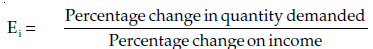There is a useful relationship between income elasticity for a goods and proportion of income spent on it. The relationship between the two is described in the following three propositions :

1. If the proportion of income spent on a goods remains the same as income increases, then income elasticity for the goods is equal to one.

2. If the proportion of income spent on a goods increases as income increases, then the income elasticity for the goods is greater than one.

3. If the proportion of income spent on a goods decreases as income rises, then income elasticity for the goods is less than one.

Income elasticity of goods reveals a few very important features of demand for the goods in question. If income elasticity is zero it signifies that the quantity demanded of the goods is quite unresponsive to changes in income. When income elasticity is greater than zero or positive then an increase in income leads to an increase in quantity demanded of the goods. This happens in case of most of the goods and such goods are called normal goods. On the other hand, goods having negative income elasticity are known as inferior goods and their demand falls as income increases. Another significant value of income elasticity is that of unity. When income elasticity of demand is equal to one, then the proportion of income spent on goods remains the same as consumer’s income increases. This represents a useful dividing line. If the income elasticity for a goods is greater than one it shows the goods bulks larger in consumer’s expenditure as he becomes richer. Such goods are called luxury goods. On the other hand, if the income elasticity is less than one it shows that the goods is relatively less important in consumer’s eye and, therefore, is called necessity.

The following examples will make the above concepts clear :

(a) The income of a household rises by 10%, the demand for wheat rises by 5%.

(b) The income of a household rises by 10%, the demand for T.V. rises by 20%.

(c) The incomes of a household rises by 5%, the demand for bajra falls by 2%.

(d) The income of a household rises by 7%, the demand for commodity X rises by 7%. (e) The income of a household rises by 5%, the demand for buttons does not change at all. Using formula for income elasticity,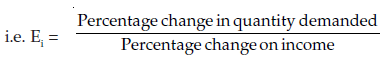we will find income-elasticity for various goods. The results are as follows :

 S. No. Commodity Income-elasticity for the household Remarks a Wheat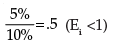since 0 < 0.5 < 1, wheat is a normal good and fulfills a necessity. b T.V.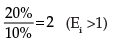since 2 > 1, T.V. is a luxurious commodity. c Bajra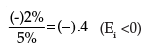since –0.4 < 0, Bajra is an inferior commodity in the eyes of household. d X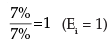since income elasticity is 1, X has unitary income elasticity. e Buttons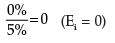Buttons have zero income-elasticity.

The various types of income elasticity explained above are in shown in the following diagram.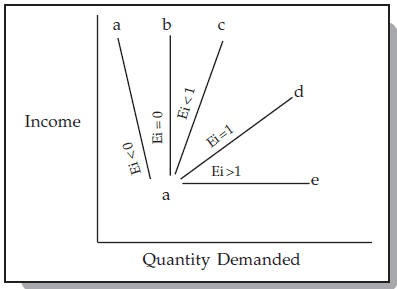Fig. 9 : Income elasticity

Income elasticity of demand will vary widely with different commodities. Generally luxuries like jewellery and fancy articles will have high income elasticity of demand, whereas ordinary household goods will have low income elasticity of demand.

It is to be noted that the words luxury, necessity, inferior goods do not signify strict dictionary meanings here. In economic theory we distinguish them in the manner shown above.

1.6.2 Cross Elasticity :

Price of Related Goods and Demand: The demand for a particular commodity may change due to the changes of prices of related goods. These related goods may be either complementary goods or substitute goods. This type of relationship is studied under ‘Cross Demand’. Cross demand refers to the quantities of a commodity or service which will be purchased with reference to changes, not of that particular commodity, but of other inter-related commodities, other things remaining the same. It may be defined as the quantities of a commodity that consumers buy per unit of time at different prices of a ‘related article’. ‘Other things remaining the same’ is the assumption which means that the income of the consumer and also the price of the commodity in question will remain constant.

Substitutes Product: In the substitute commodities the cross demand curve slopes upwards (i.e. is positive) showing that more quantities of a commodity will be demanded whenever there is a rise in price of a substitute commodity. In the figure, quantity demanded of Tea is given on the X axis. Y axis represents the price of coffee which is a substitute for a tea. When the price of coffee increases, the demand for coffee becomes less due to the operation of the law of demand. But the consumers will go in for ‘tea’ to substitute in the place of coffee. The price of tea is assumed to be constant. So whenever there is an increase in price of one commodity, the demand for the substitute commodity will increase.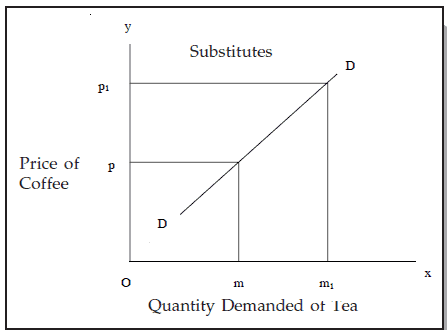Fig. 10 : Substitutes

Complementary Goods

In the case of complementary goods, as shown in the figure, a change in price of a good will have an opposite reaction on the demand of other commodity which is closely related or complementary. For instance, an increase in demand for pen will necessarily increase the demand for ink; so also bread and butter; horse and carriages, etc. Whenever there is a fall in demand of fountain pens due to the rise in prices of fountain pens, the demand for ink will fall down, not that the price of ink has gone up, but because the price of fountain pen has gone up. So we find that there is an inverse relationship between price of a commodity and demand for its complementary good (other things remaining the same).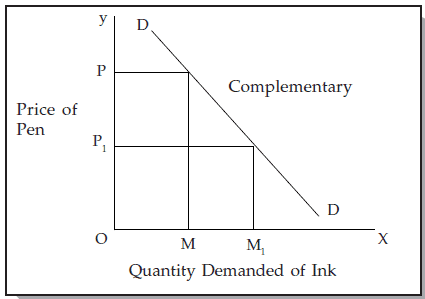Fig. 11 : Complementary Goods

A change in the demand for one goods in response to a change in the price of another goods represents cross elasticity of demand of the former goods for the latter goods. Symbolically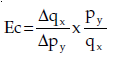Where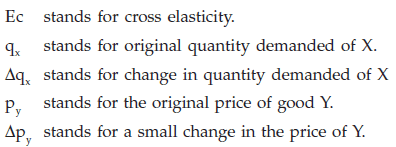If two goods are perfect substitutes for each other cross elasticity is infinite and if two goods are totally unrelated, cross elasticity between them is zero.

If the two goods are substitutes (like tea and coffee) the cross elasticity is positive, that is, in response to a rise in price of one goods the demand for the other goods rises. On the other hand, when two goods are complementary (tea and sugar) to each other, the cross elasticity between them is negative so that a rise in the price of one leads to a fall in the quantity demanded of the other. However, one need not base the classification of goods on the above definitions. While the goods between which cross elasticity is positive can be called substitutes, the goods between which cross elasticity is negative are not always complementary. This is because negative cross elasticity is also found when the income effect on the price change is very strong.

1.7 DEMAND DISTINCTIONS

Certain important demand distinctions are as follows:
a. Producers goods and Consumer’s goods
b. Durable goods and Non-durable goods
c. Derived demand and Autonomous demand
d. Industry demand and Company demand
e. Short-run demand and Long-run demand

a. Producer’s goods and Consumer’s goods: Producer’s goods are those which are used for the production of other goods- either consumer goods or producer goods themselves. Examples of such goods are machines, locomotives, ships etc. Consumer’s goods are those which are used for final consumption. Examples of consumer’s goods can be readymade clothes, prepared food, residential houses, etc.

b. Durable goods and Non-durable goods: Consumer’s goods may be further sub-divided into durable and non-durable goods. The non-durable consumer goods are those which cannot be consumed more than once; for example bread, milk etc. These will meet only the current demand. On the other hand, durable consumer goods are those which can be consumed more than once over a period of time, example, a car, a refrigerator, a ready-made shirt, and umbrella. The demand for durable goods is likely to be a derived demand.

c. Derived demand and Autonomous demand:  When a product is demanded consequent on the purchase, of a parent product, its demand is called derived demand. For example, the demand for cement is derived demand, being directly related to building activity. If the demand for a product is independent of demand for other goods, then it is called autonomous demand. But this distinction is purely arbitrary and it is very difficult to find out which product is entirely independent of other products.

d. Industry demand and Company demand:  The term industry demand is used to denote the total demand for the products of a particular industry, e.g. the total demand for steel in the country. On the other hand, the term company demand denotes the demand for the products of a particular company, e.g. demand for steel produced by the Tata Iron and Steel Company.

e. Short –run demand and Long-run: demand Short run demand refers to demand with its immediate reaction to price changes, income fluctuations, etc., whereas long-run demand is that which will ultimately exists as a result of the changes in pricing, promotion or product improvement, after enough time is allowed to let the market adjust to the new situation. For example, if electricity rates are reduced, in the short run, the existing users will make greater use of electric appliances. In the long run more and more people will be induced to use electric appliances.

SUMMARY

An individual’s demand for a product depends upon the price of the product, income of the individual and the prices of related goods. But amongst these determinants of demand, economists single out price of the goods in question as the most important factor governing the demand for it. Indeed, the function of a theory of demand is to establish a relationship between price and the quantity demanded of a goods and to provide explanation for it. This relationship is illustrated graphically by a demand curve that shows how much will be demanded at each market price.

The demand curve will shift to right by a rise in income (unless the goods is an inferior one), a rise in the price of a substitute, a fall in the price of a complement, a rise in population and a change in tastes in favour of this commodity. The opposite changes will shift the demand curve to the left. As against these when the price of the commodity rises, the consumer goes up the demand curve and when the price falls, consumer goes down the demand curve.

Price elasticity of demand is a measure of the extent to which the quantity demanded of a goods responds to a change in its price. When the numerical measure is less than one, we say that the demand is inelastic when it is greater than one, we say demand is elastic and when it is equal to one we say demand is unitary. Two special cases are when elasticity equals zero or infinity. When elasticity is equal to zero, the quantity demanded does not change at all as price changes, and when elasticity equals infinity, a very small reduction in price increases the quantity demanded from zero to an infinitely large number. Price elasticity can be measured at a point or between two points. Here we use the concepts of point elasticity and arc elasticity respectively. The main determinants of elasticity are the availability of substitutes for the commodity, number of uses of the commodity, nature of commodity, etc.

Income elasticity measures the response of quantity demanded to a percentage change in income of the consumer. Cross elasticity is the percentage change in quantity demanded of a product as a result of change in the price of its related product.

Offer running on EduRev: Apply code STAYHOME200 to get INR 200 off on our premium plan EduRev Infinity!

## Economics for CA CPT

66 videos|78 docs|59 tests

,

,

,

,

,

,

,

,

,

,

,

,

,

,

,

,

,

,

,

,

,

;